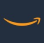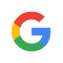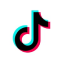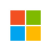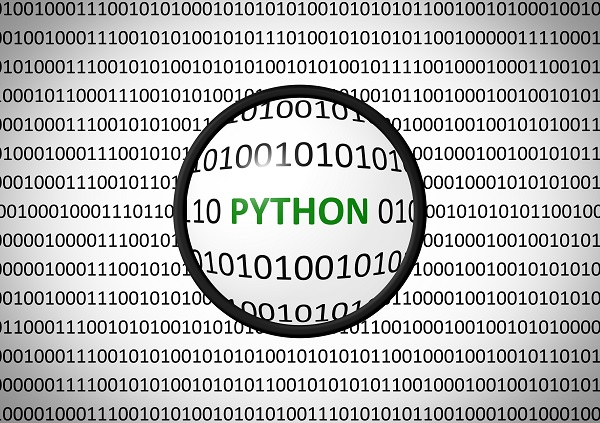How to learn matplotlib, numpy, scipy, pandas in Python systematically?
Summarize my learning, confronted with Numpy, Pandas, Matplotlib, Scipy, and Scikit-learn, which is also considered an entry-level, give my own trajectory (omitted installation), and summarize the answers of others, and finally, there are painted eggshell.

Numpy：

is used to store and process large matrix, much more efficient than Python's own nested list (nested list structure) structure, Itself is developed by C language. This is a very basic extension, the rest of the extensions are based on it. The data structure is an ndarray, and there are generally three ways to create it.

1. Python object transformation

2. Generated by similar factory functions numpy built-in functions: np.range,np.linspace.....

Pandas:

A tool based on NumPy was created to solve data analysis tasks. Pandas incorporate a large number of libraries and some standard data models, providing the tools necessary to manipulate large data sets efficiently. The most statistically significant toolkit, superior to the R software in some respects. Data structures include one-dimensional Series, two-dimensional DataFrame (similar to Excel or SQL tables, if you study in-depth, you will find a lot of similarities between Pandas and SQL, such as merge function), three-dimensional Panel (Pan(el) + da(ta) + s, you know where the name comes from). To learn Pandas you need to master the following：

1. summarize and calculate descriptive statistics, handle missing data , hierarchical indexing

2. cleanup, conversion, merge, reshape, GroupBy techniques

3. date and time data types and tools (date processing is very convenient)

Matplotlib:

Python's most famous drawing system, and many other drawing systems, such as seaborn (for pandas drawing) are wrapped in it. The creator, John Hunter, passed away in 2012. This plotting system is very complex, making it seem prohibitive compared to R's ggplot and lattice plots, which is why I don't discard R, although using the plotted graphs can be displayed roughly in the colors of ggplot,  it still feels like chicken ribs. But the complexity of matplotlib gives it strong customizability. It has an object-oriented approach and Pyplot's classic high-level encapsulation.

What you need to master are:

1. scatter diagram, line chart, bar chart, histogram, pie chart, box plot drawing.

2. three major systems of plotting: pyplot, pylab (not recommended), object-oriented

3. coordinate axis adjustment, add text annotations, region filling, and the use of special graphics patches

4. financial students note that: you can directly call Yahoo financial data plotting

Scipy:

A convenient, easy-to-use Python toolkit designed for science and engineering. It includes statistics, optimization, integration, linear algebra modules, Fourier transformation, signal and image processing, normal differential equation solvers, and more.

Basically, it can replace Matlab, However, if you use it, it has very few relationships with data processing, the Department of Mathematics, or Engineering Department is relatively more used. (omitted)

Recently found a statsmodel can supplement scipy.stats, time series support perfect

Scikit-learn：

Students who are concerned about machine learning can pay attention to the very hot open source machine learning tools, there are many in this area, such as TensorFlow open-source of Google at the end of last year, or Theano, caffe (Jia Yangqing), Keras and so on, which are another aspects of the problems.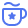直连行业大牛导师，1v1模拟面试与求职指导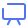实战与求职精品课程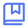2000+名企面试真题